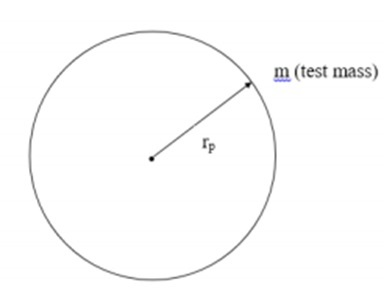# Hubble Parameter & Scale Factor

In this chapter, we will discuss regarding the Hubble Parameter as well as the Scale Factor.

• Prerequisite − Cosmological Redshift, Cosmological Principles.

• Assumption − The universe is homogenous and isotropic.

## Hubble’s Constant with Fractional Rate of Change of Scale Factor

In this section, we will relate the Hubble’s Constant with fractional rate of Change of Scale Factor.

We can write velocity in the following manner and simplify.

$$v = \frac{\mathrm{d} r_p}{\mathrm{d} t}$$

$$= \frac{d[a(t)r_c}{dt}$$

$$v = \frac{\mathrm{d} a}{\mathrm{d} t} \ast \frac{1}{a} \ast (ar_c)$$

$$v = \frac{\mathrm{d} a}{\mathrm{d} t} \ast \frac{1}{a} \ast r_p$$

Here, v is the recessional velocity, a is the scale factor and rp is the proper distance between the galaxies.

Hubble’s Empirical Formula was of the nature −

$$v = H \ast r_p$$

Thus, comparing the above two equations we obtain −

Hubble’s Parameter = Fractional rate of change of the scale factor

$$H = da/dt \ast 1/a$$

Note − This is not a constant since the scale factor is a function of time. Hence it is called the Hubble’s parameter and not the Hubble’s constant.

Empirically we write −

$$H = V/D$$

Thus, from this equation, we can infer that since D is increasing and V is a constant, then H reduces with the time and expansion of the universe.

## Friedmann Equation in Conjunction with the Robertson-Walker Model

In this section, we will understand how the Friedmann Equation is used in conjunction with the Robertson-Walker model. To understand this, let us take the following image which has a test mass at distance rp from body of mass M as an example.Taking into consideration the above image, we can express force as −

$$F = G \ast M \ast \frac{m}{r^2_p}$$

Here, G is the universal gravitational constant and ρ is the matter density inside the observable universe.

Now, assuming uniform mass density within the sphere we can write −

$$M = \frac{4}{3} \ast \pi \ast r_p^3 \ast \rho$$

Using these back in our force equation we get −

$$F = \frac{4}{3} \ast \pi \ast G \ast r_p \ast \rho \ast m$$

Thus, we can write the potential energy and kinetic energy of the mass m as −

$$V = -\frac{4}{3} \ast \pi \ast G \ast r^2_p \ast m \ast \rho$$

$$K.E = \frac{1}{2} \ast m \ast \frac{\mathrm{d} r_p^2}{\mathrm{d} t}$$

Using the Virial Theorem

$$U = K.E + V$$

$$U = \frac{1}{2} \ast m \ast \left ( \frac{\mathrm{d} r_p}{\mathrm{d} t} \right )^2 - \frac{4}{3} \ast \pi \ast G \ast r_p^2 \ast m \ast \rho$$

But here, $r_p = ar_c$. So, we get −

$$U = \frac{1}{2} \ast m \ast \left ( \frac{\mathrm{d} a}{\mathrm{d} t} \right )^2 r_c^2 - \frac{4}{3} \ast \pi \ast G \ast r_p^2 \ast m \ast \rho$$

On further simplification, we obtain the Friedmann equation,

$$\left ( \frac{\dot{a}}{a} \right )^2 = \frac{8\pi}{3} \ast G \ast \rho + \frac{2U}{m} \ast r_c^2 \ast a^2$$

Here U is a constant. We also note that the universe we live in at present is dominated by matter, while the radiation energy density is very low.

### Points to Remember

• The Hubble parameter reduces with time and expansion of the universe.

• The universe we live in at present is dominated by matter and radiation energy density is very low.